# Normal distribution facts for kids

Kids Encyclopedia Facts
(Redirected from Normal Distribution)
Parameters Probability density function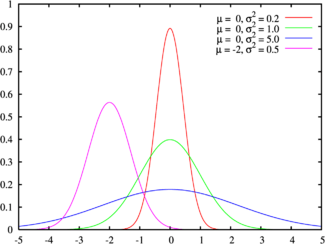The green line is the standard normal distribution Cumulative distribution function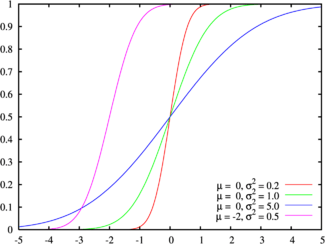Colors match the image above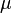$\mu$ location (real)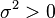$\sigma^2>0$ squared scale (real)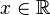$x \in\mathbb{R}\!$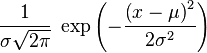$\frac1{\sigma\sqrt{2\pi}}\; \exp\left(-\frac{\left(x-\mu\right)^2}{2\sigma^2} \right) \!$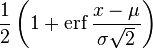$\frac12 \left(1 + \mathrm{erf}\,\frac{x-\mu}{\sigma\sqrt2}\right) \!$$\mu$$\mu$$\mu$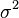$\sigma^2$ 0 0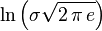$\ln\left(\sigma\sqrt{2\,\pi\,e}\right)\!$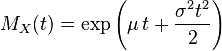$M_X(t)= \exp\left(\mu\,t+\frac{\sigma^2 t^2}{2}\right)$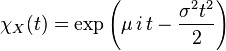$\chi_X(t)=\exp\left(\mu\,i\,t-\frac{\sigma^2 t^2}{2}\right)$

The normal distribution is a probability distribution. It is also called Gaussian distribution because it was discovered by Carl Friedrich Gauss. The normal distribution is a continuous probability distribution. It is very important in many fields of science. Normal distributions are a family of distributions of the same general form. These distributions differ in their location and scale parameters: the mean ("average") of the distribution defines its location, and the standard deviation ("variability") defines the scale.

The standard normal distribution (also known as the Z distribution) is the normal distribution with a mean of zero and a variance of one (the green curves in the plots to the right). It is often called the bell curve because the graph of its probability density looks like a bell.

Many values follow a normal distribution. This is because of the central limit theorem, which says that if an event is the sum of other random events, it will be normally distributed. Some examples include:

• Measurement errors
• Light intensity (so-called Gaussian beams, as in laser light)
• Intelligence is probably normally distributed. There is a problem with accurately defining or measuring it, though.
• Insurance companies use normal distributions to model certain average cases.

## Images for kidsNormal distribution Facts for Kids. Kiddle Encyclopedia.